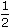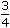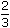# Aptitude - Stocks and Shares

Exercise : Stocks and Shares - General Questions
1.
In order to obtain an income of Rs. 650 from 10% stock at Rs. 96, one must make an investment of:
Rs. 3100
Rs. 6240
Rs. 6500
Rs. 9600
Explanation:

To obtain Rs. 10, investment = Rs. 96.

 To obtain Rs. 650, investment = Rs.96 x 650= Rs. 6240. 10

2.
A man bought 20 shares of Rs. 50 at 5 discount, the rate of dividend being 13. The rate of interest obtained is:
 12 1 % 2
 13 1 % 2
15%
 16 2 % 3
Explanation:

Investment = Rs. [20 x (50 - 5)] = Rs. 900.

Face value = Rs. (50 x 20) = Rs. 1000.

 Dividend = Rs.27 x 1000= Rs. 135. 2 100

 Interest obtained =135 x 100% = 15% 900

3.
Which is better investment: 11% stock at 143  or  9% stock at 117?
11% stock at 143
 9 3 % stock at 117 4
Both are equally good
Cannot be compared, as the total amount of investment is not given.
Explanation:

Let investment in each case be Rs. (143 x 117).

 Income in 1st case = Rs.11 x 143 x 117= Rs. 1287. 143

 Income in 2nd case = Rs.39 x 143 x 117= Rs. 1394.25 4 x 117

 Clearly, 9 3 % stock at 117 is better. 4

4.
A man buys Rs. 20 shares paying 9% dividend. The man wants to have an interest of 12% on his money. The market value of each share is:
Rs. 12
Rs. 15
Rs. 18
Rs. 21
Explanation:

 Dividend on Rs. 20 = Rs.9 x 20= Rs. 9 . 100 5

Rs. 12 is an income on Rs. 100.Rs. 9 is an income on Rs.100 x 9= Rs. 15. 5 12 5

5.
By investing in 16% stock at 64, one earns Rs. 1500. The investment made is:
Rs. 5640
Rs. 5760
Rs. 7500
Rs. 9600
 To earn Rs. 1500, investment = Rs.64 x 3 x 1500= Rs. 5760. 50